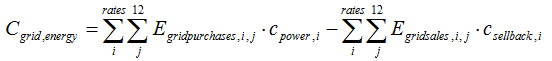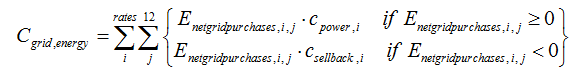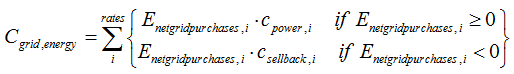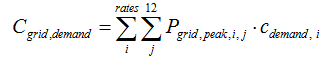# HOMER Pro 3.11

 Navigation: Results > Simulation Results Grid OutputsThe Grid tab in the Simulation Results window displays details about the buying and selling of electricity from and to the grid, and the resulting costs. The outputs table contains the following variables.

 Variable Description Energy Purchased The total amount of electricity purchased from the grid, in kWh Energy Sold The total amount of electricity sold to the grid, in kWh Net Energy Purchased The net electricity purchased from the grid, in kWh Peak Demand The peak power demand serviced by the grid, in kW Energy Charge The total amount paid in energy charges, in \$ Demand Charge The total amount paid in demand charges, in \$

If you defined more than one rate in the Grid Inputs on the Grid page, the Rate Schedule drop-down menu allows you to choose a rate, or select All to see the summation over all rates.

## Energy Charge

If net metering does not apply, HOMER calculates the total annual energy charge using the following equation:where: Egrid-purchases,i,j = the amount of energy purchased from the grid in month j during the time that rate i applies [kWh] cpower,i = the grid power price for rate i [\$/kWh] Egrid-sales,i,j = the amount of energy sold to the grid in month j during the time that rate i applies [kWh] csellback,i = the sellback rate for rate i [\$/kWh]

If net metering applies and net generation is calculated monthly, HOMER calculates the total annual energy charge using the following equation:where: Enet-grid-purchases,i,j = the net grid purchases (grid purchases minus grid sales) in month j during the time that rate i applies [kWh] cpower,i = the grid power price for rate i [\$/kWh] csellback,i = the sellback rate for rate i [\$/kWh]

If net metering applies and net generation is calculated annually, HOMER calculates the total annual energy charge using the following equation:where: Enetgridpurchases,i = the annual net grid purchases (grid purchases minus grid sales) during the time that rate i applies [kWh] cpower,i = the grid power price for rate i [\$/kWh] csellback,i = the sellback rate for rate i [\$/kWh]

## Demand Charge

HOMER calculates the total annual (listed after December) grid demand charge using the following equation:where: Pgrid,peak,i,j = the peak hourly grid demand in month j during the time that rate i applies [kWh] cdemand,i = the grid demand rate for rate i [\$/kW/month]